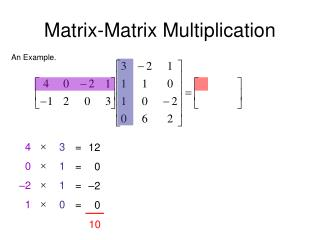DownloadDownload PresentationMatrix-Matrix Multiplication

# Matrix-Matrix Multiplication

Télécharger la présentation## Matrix-Matrix Multiplication

- - - - - - - - - - - - - - - - - - - - - - - - - - - E N D - - - - - - - - - - - - - - - - - - - - - - - - - - -
##### Presentation Transcript

1. Matrix-Matrix Multiplication An Example.     4 0 –2 1 3 1 1 0 = = = = 12 0 –2 0 10

2. Matrix-Matrix Multiplication An Example.     –1 2 0 3 3 1 1 0 = = = = –3 2 0 0 –1

3. Matrix-Matrix Multiplication An Example.     4 0 –2 1 –2 1 0 6 = = = = –8 0 0 6 –2

4. Matrix-Matrix Multiplication An Example.     –1 2 0 3 –2 1 0 6 = = = = 2 2 0 18 22

5. Matrix-Matrix Multiplication An Example.     4 0 –2 1 1 0 –2 2 = = = = 4 0 4 2 10

6. Matrix-Matrix Multiplication An Example.     –1 2 0 3 1 0 –2 2 = = = = –1 0 0 6 5

7. Matrix-Matrix Multiplication An Example.

8. Matrix-Matrix Multiplication Another Example. 2x + 3y – 2z –4x + 2y + 3z 5x + 7y + 6z This gives us a simple and elegant way to write a system of linear equations. 2x + 3y – 2z = –2 –4x + 2y + 3z = 1 5x + 7y + 6z = 28 Then the system can be written as

9. Properties Real-number multiplication is commutative. No! Is matrix multiplication commutative? Real-number multiplication is associative. Yes! Is matrix multiplication commutative? Real-number multiplication has an identity. Yes! Does matrix multiplication have an identity? (but you must use an identity matrix of the proper size for A) Real-number multiplication has inverses. Unless a = 0. Does matrix multiplication have an identity? Yes! Unless det(A) = 0.

10. Find:

11. Matrices: Inverses (and Identities) Matrix Equations A system of equations can be written quite simply as AX = C. To solve it like a real-number equation, we will need the multiplicative identity and inverse for matrices. Multiplicative inverses multiply to the multiplicative identity. The multiplicative identity disappears from multiplication. We will have solved the equation.

12. Matrices: Inverses (and Identities) • Finding The Inverse Of A Matrix • The General Procedure to find the inverse of A. • Write a rectangular augmented matrix [ A | I ]. • Use Gauss-Jordan elimination (row operations on the whole matrix) to change A into the identity. You will now have [ I | A–1 ]. I can demonstrate this process quickly online here. If that link doesn’t work right, try this one and use the “row operation calculator.” A matrix A that has no inverse (because det(A) = 0) is called a singular matrix. A matrix A that has an inverse (because det(A)  0) is called a nonsingular matrix